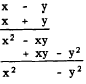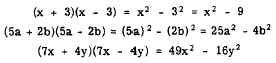Multiplication of a polynomial by a polynomialCustom SearchMULTIPLICATION OF A POLYNOMIAL BY A POLYNOMIAL As with the monomial multiplier, we explain multiplication of a polynomial by a polynomial by use of an arithmetic example. To multiply (3 + 2)(6 - 4), we could do the operation within the parentheses first and then multiply, as follows: (3 + 2)(6 - 4) = (5)(2) = 10 However, thinking of the quantity (3 + 2) as one term, we can use the method described for a (3 + 2), with the following result: (3 + 2)(6 - 4) = [(3 + 2) x 6 - (3 + 2) x 4] Now considering each of the two resulting products separately, we note that each is a binomial multiplied by a monomial. The first is (3 + 2)6 = (3 x 6) + (2 x 6) and the second is -(3 + 2)4 = - [(3 x 4) + (2 x 4)]                 = -(3 x 4) - (2 x 4) Thus we have the following result: (3 + 2)(6 - 4) = (3 X 6) + (2 X 6)                          -(3 x 4) - (2 x 4)                        = 18 + 12 - 12 - 8                        = 10 The complete product is formed by multiplying each term of the multiplicand separately by each term of the multiplier and combining the results with due regard to signs. Now let us apply this method in two examples involving literal numbers. 1. (a + b)(m + n) = am + an + bm + bn 2. (2b + c)(r + s + 3t - u) = 2br + 2bs + 6bt - 2bu + cr + cs + 3ct - cu The rule governing these examples is stated as follows: The product of any two polynomials is found by multiplying each term of one by each term of the other and adding the results algebraically. It is often convenient, especially when either of the expressions contains more than two terms, to place the polynomial with the fewer terms beneath the other polynomial and multiply term by term beginning at the left. Like 3x2 - 7x - 9 and 2x - 3. The procedure isPractice problems. In the following problems, multiply and combine like terms:SPECIAL PRODUCTS The products of certain binomials occur frequently. It is convenient to remember the form of these products so that they can be written immediately without performing the complete multiplication process. We present four such special products as follows, and then show how each is derived: 1. Product of the sum and difference of two numbers. EXAMPLE: (x - y)(x + y) = x2 - y2 2. Square the sum of two numbers. EXAMPLE: (x+y)2=x2 +2xy+y2 3. Square of the difference of two numbers. EXAMPLE: (x - Y)2 = x2 -2xy+y2 4. Product of two binomials having a common term. EXAMPLE: (x + a)(x + b) = x2 + (a + b)x + ab Product of Sum and Difference The product of the sum and difference of two numbers is equal to the square of the first number minus the square of the second number. If, for example, x - y is multiplied by x + y, the middle terms cancel one another. The result is the square of x minus the square of y, as shown in the following illustration:By keeping this rule in mind, the product of the sum and difference of two numbers can be written down immediately by writing the difference of the squares of the numbers. For example, consider the following three problems:Integrated Publishing, Inc. - A (SDVOSB) Service Disabled Veteran Owned Small Business